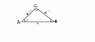# Two Solutions for Trig Functions: An Intuitive Way

• 462chevelle
In summary, you can use the Law of Sines to find the angles between two sides of a triangle. If one angle is given, you can find the sin of the other angle. If the angle opposite to the longer side is given, you can find the sin of the angle opposite to the shorter side. There exist two angles between 0 and 180 ° with the same sin.f

#### 462chevelle

Gold Member
when working with trig functions. is there a trick to knowing if there are 2 solutions while filling out a triangle without memorizing your sines and cosines. or do I need to be subtracting all numbers I get by 180 then sin/cos them to see if they have the same number? doing law of sines right now. I would like to make it intuitive somehow without chasing rabbits on every problem if there is a way. thanks I attempted to attach a pic of the problem.
using the law of sines I got C is 50 and B is 72. rounding to the tens place for simplicity. this is just independent study not for a class. but my issue is the answer has 2 answers. I understand why. but I am curious if there is a way to tell without plugging numbers in and trying.

thanks

#### Attachments

•trig pic.jpg
5.2 KB · Views: 393
You need a problem statement - I cannot see where you get two answers if there is no question.

You can draw a single triangle if given the lengths of two sides and the angle opposite to the longer side. But given the angle opposite to the shorter side, you can draw two triangles. Try.

ehild

Hmmm... in the example, the angle opposite the shorter side is given.
There are two places that side a can connect to side c, giving two possible lengths for c - and two possible angles for C and B.

Hmmm... in the example, the angle opposite the shorter side is given.
There are two places that side a can connect to side c, giving two possible lengths for c - and two possible angles for C and B.

so what method should I use for finding the second set of angles? taking B and doing 180-b and that's my second possible angle for B??

You know know how to identify the situation where you'll get two possible answers.
Practice drawing out a few of these and see what the relationships are.

Per your example, c can be long, as drawn, or very short.
Have a look at the two possibilities for angle B. If one is ##\small \theta## then, yep, the other is ##\small 180-\theta##.
Once you have B, the triangle has only one solution.

now it seems like that was something I should have realized without help. I guess I need to think about it in a little broader sense before I throw in the towel and ask. thanks for the explanation.

It's easy to miss - most people don't think of constructing triangles - you normally just sketch them out.

ya I still have a bad habit of just drawing every triangle and marking it from left bottom point AbCaBc. and not drawing to scale. but I am still not a master of the relationships between the sides length and the angles without the math. guess that's why math requires so much practice.

The Law of Sines states that a/b = sinα /sinβ, where α is the angle opposite to a and β is opposite to b. If a>b α>β.

If one angle is given, you get the sin of the other angle, sinβ= sinα b/a or sinα= sinβ a/b and there exist two angles between 0 and 180 ° with the same sine.

If the angle opposite to the longer side (a) is given the other angle must be smaller than it. It can be obtuse only if α was obtuse, but a triangle can not have two obtuse angles. So β must be an acute angle: there is only one angle with the given sinβ=sinα b/a

If the angle β, opposite to the shorter side is given, α>β, sinα=sinβ a/b < 1, there are two possible angles with the same sine, one acute and the other obtuse; they are supplementary angles.

ehild

Last edited: